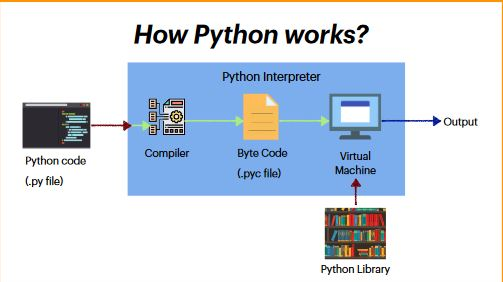# What is the difference between .py and .pyc file

Published February 04, 2021

We all know we will save the Python files with .py. This .py file contains the actual source code of the each python program.

The More about the Python .py and .pyc files are below

1. .py files contain the source code of a program. Whereas, .pyc file contains the bytecode of your program. We get bytecode aftercompilation of .py file (source code). .pyc files are not created for all the files that you run. It is only created for the files that you import.
2. Before executing a python program python interpreter checks for the compiled files. If the file is present, the virtual machine executes it. If not found, it checks for .py file. If found, compiles it to .pyc file and then python virtual machine executes it.
3. Having .pyc file saves you the compilation time

How Python works?Simple Python Example

 def recursive_sum(n):     if n <= 1:         return n     else:         return n + recursive_sum(n-1) # change this value for a different result number = 22 if number < 0:     print("Enter a positive number") else:     print("The sum is",recursive_sum(number))

Output

```\$python3 main.py
The sum is 253```

If we store this program with the name "sample",then it will be stored as "sample.py" when we run a sample.py file, compiler  creates a sample.pyc file once the code is executed it first executes .py file where as code is first turned into byte code by the compiler in the form of "sample.pyc” file

 Article Contributed By :2166 Views